Question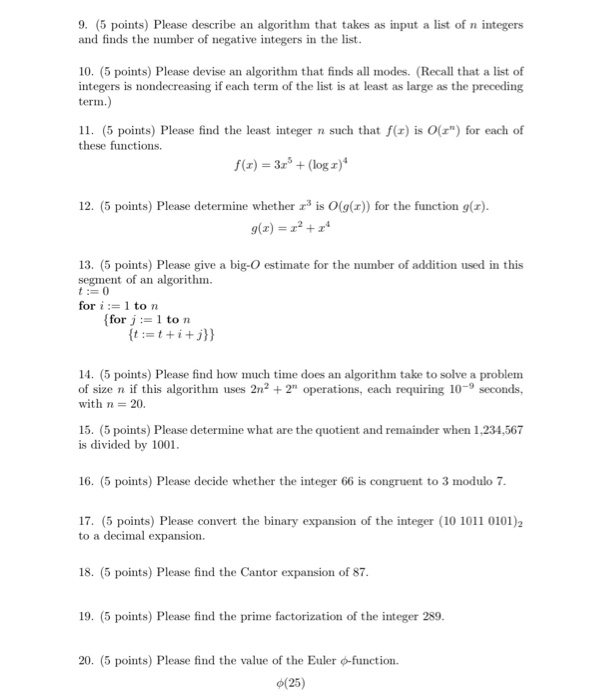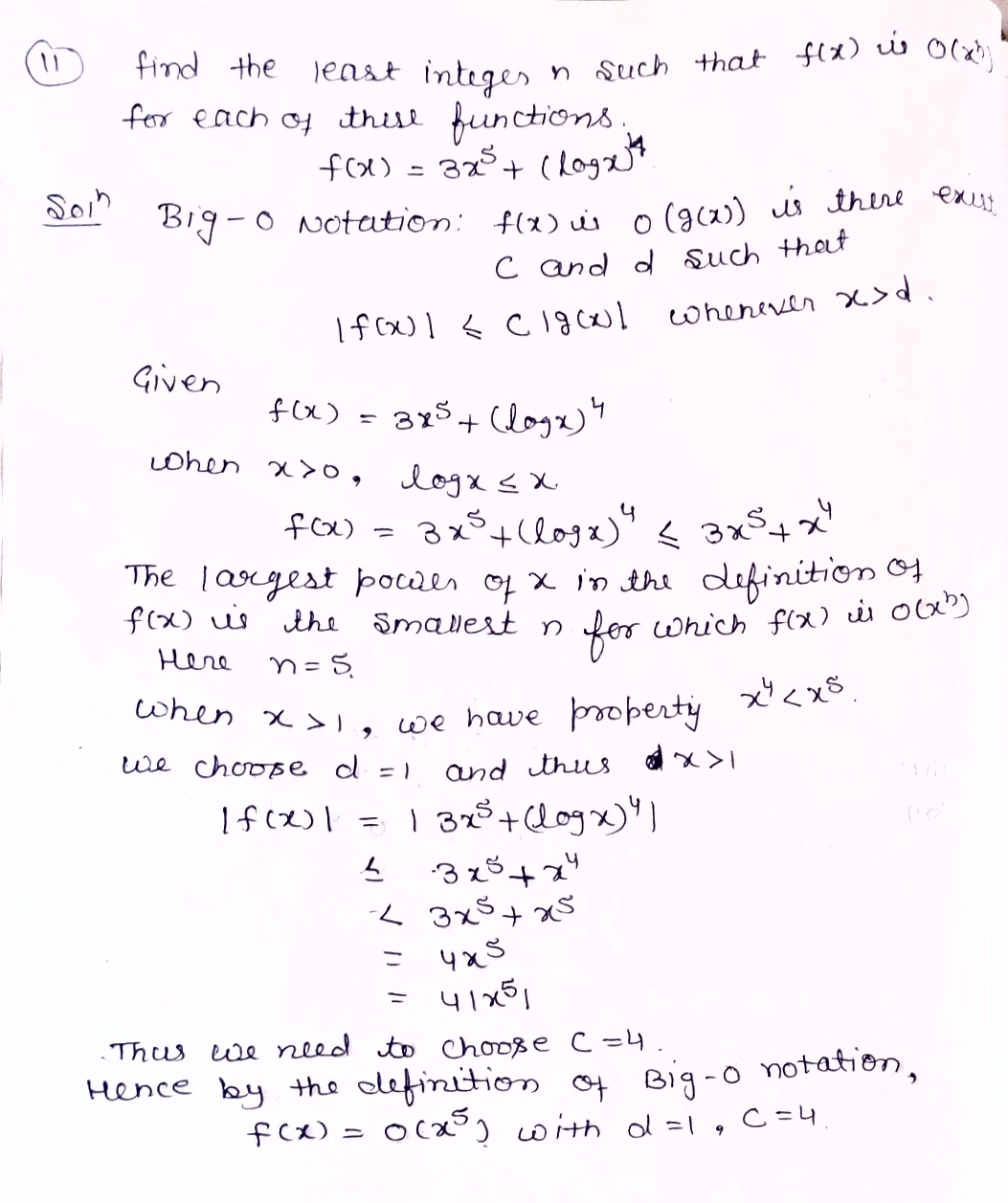#### Earn Coins

Coins can be redeemed for fabulous gifts.

Similar Homework Help Questions
• ### Describe an algorithm that takes as input a list of n integers and produces output the...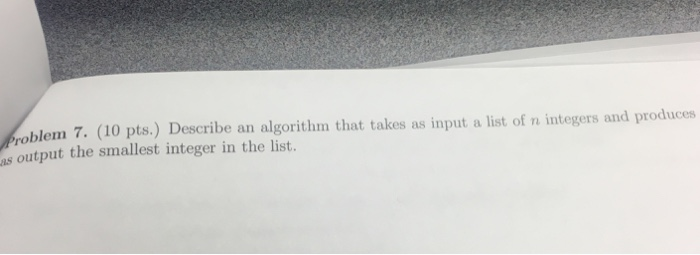Describe an algorithm that takes as input a list of n integers and produces output the smallest integer in the list.

• ### a. Use pseudocode to specify a brute-force algorithm that takes as input a list of n...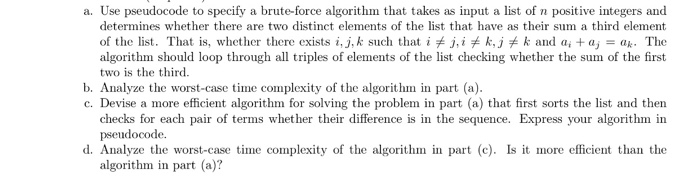a. Use pseudocode to specify a brute-force algorithm that takes as input a list of n positive integers and determines whether there are two distinct elements of the list that have as their sum a third element of the list. That is, whether there exists i, j.k such that iヂj, i关k,j关k and ai + aj = ak. The algorithm should loop through all triples of elements of the list checking whether the sum of the first two is the third...

• ### Problem 3: (5 2 points) Design an algorithm that takes an array of positive integers A...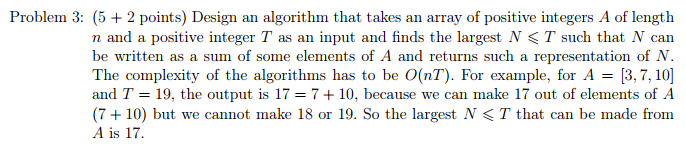Problem 3: (5 2 points) Design an algorithm that takes an array of positive integers A of length n and a positive integer T as an input and finds the largest N < T such that N can be written as a sum of some elements of A and returns such a representation of N. The complexity of the algorithms has to be O(nT). For example, for A 3,7, 10 and T 19, the output is 17 7+10, because we...

• ### Describe a non-recursive algorithm that takes a list of distinct integers a_1, a_2, ...., a_n and...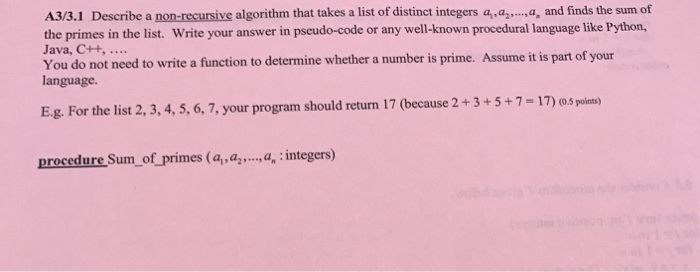Describe a non-recursive algorithm that takes a list of distinct integers a_1, a_2, ...., a_n and finds the sum of the primes in the list. Write your answer in pseudo-code or any well-known procedural language like Python, Java, C++, ..... You do not need to write a function to determine whether a number is prime. Assume it is part of your language. E.g. For the list 2, 3, 4, 5, 6, 7, your program should return 17 (because 2 +...

• ### 1 1 point Consider the following algorithm for factoring an integer N provided as input (in...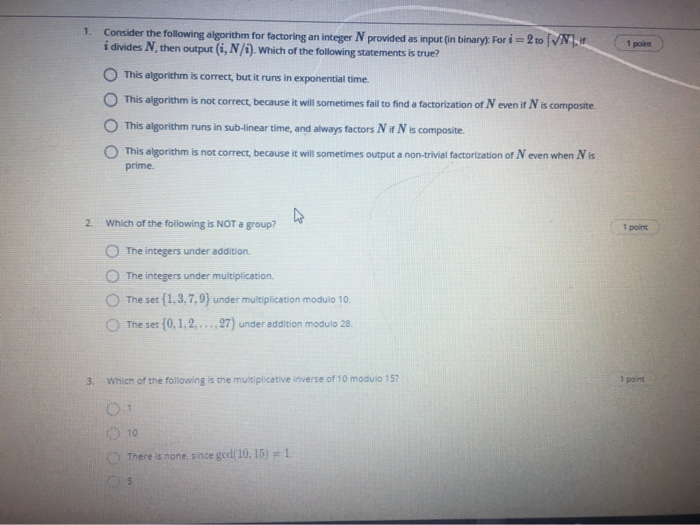1 1 point Consider the following algorithm for factoring an integer N provided as input (in binary): For i = 2 to [VN.17 i divides N, then output (i, N/). Which of the following statements is true? This algorithm is correct, but it runs in exponential time. This algorithm is not correct, because it will sometimes fail to find a factorization of Neven if N is composite This algorithm runs in sub-linear time, and always factors N it Nis composite...

• ### 8. Consider the following algorithm, which finds the sum of all of the integers in a...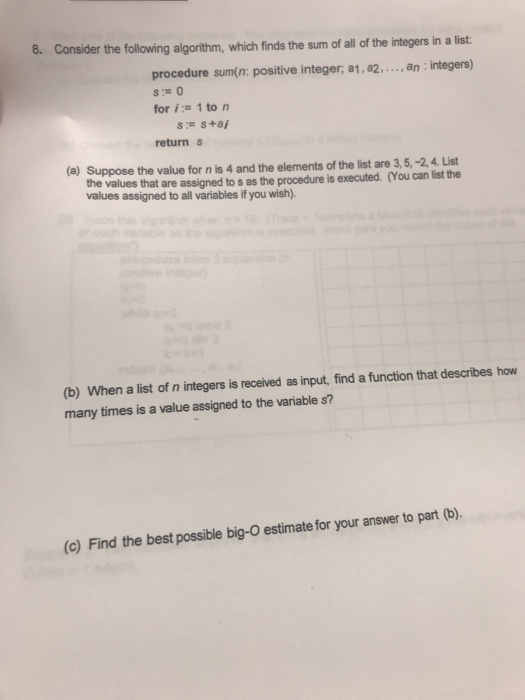8. Consider the following algorithm, which finds the sum of all of the integers in a list procedure sum(n: positive integer, a1, a2,..., an : integers) for i: 1 to n return S (a) Suppose the value for n is 4 and the elements of the list are 3, 5,-2,4. List assigned to s as the procedure is executed. (You can list the the values that are values assigned to all variables if you wish) b) When a list of...

• ### 5. (570/470 bonus) Design an algorithm whose input is a list of n points, (xu, )...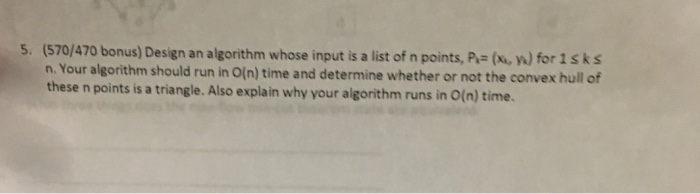5. (570/470 bonus) Design an algorithm whose input is a list of n points, (xu, ) for isks n. Your algorithm should run in O(n) time and determine whether or not the convex hull of these n points is a triangle. Also explain why your algorithm runs in O(n) time.

• ### 9. [10 points) Consider the following algorithm: procedure Algorithm(n: positive integer; ddd: distinet integers) for k:=1 to n-1 for 1-1 to n-k print(k, I, di,da...-1,dn) if ds dti then interch...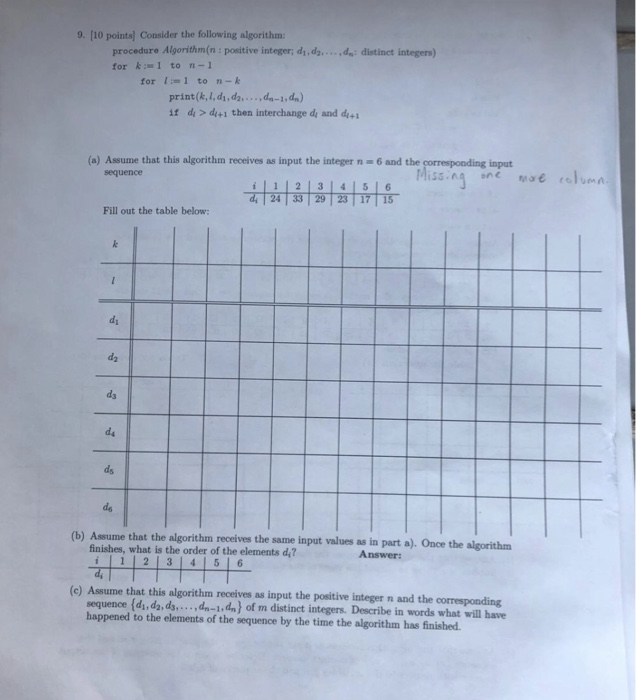9. [10 points) Consider the following algorithm: procedure Algorithm(n: positive integer; ddd: distinet integers) for k:=1 to n-1 for 1-1 to n-k print(k, I, di,da...-1,dn) if ds dti then interchange dy and d (a) Assume that this algorithm receives as input the integer n 6 and the input sequence 하하하하하하, Miss ^-ruteae rehen i12|3141516 Fill out the table below: ds ds (b) Assume that the algorithm receives the same input values as in part a). Once the algorithm finishes, what...

• ### 8. [10 points) Consider the following algorithm procedure Algorithm(: integer, n: positive integer; 81,...a s integers with vhilei<r print (l, r, mı, arn, 》 if z > am then 1:= m + 1 if za...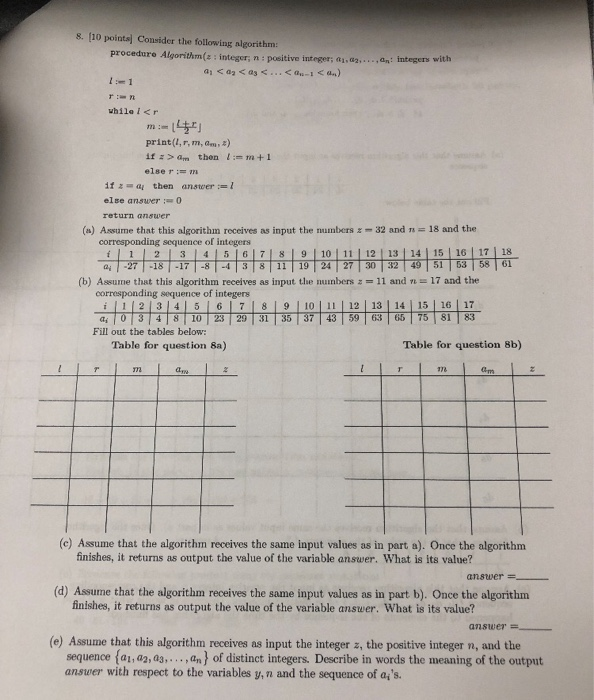8. [10 points) Consider the following algorithm procedure Algorithm(: integer, n: positive integer; 81,...a s integers with vhilei<r print (l, r, mı, arn, 》 if z > am then 1:= m + 1 if za then anstwer-1 return answer 18 and the (a) Assume that this algorithm receives as input the numbersz-32 and corresponding sequence of integers 2 | 3 1 1 4151617| 8| 9 | 10 İ 11 İ 12 | 13 | 14|15 | 16 | 17 |...

• ### (15 points) Consider the algorithm for insertion sort shown below. The input to this algorithm is...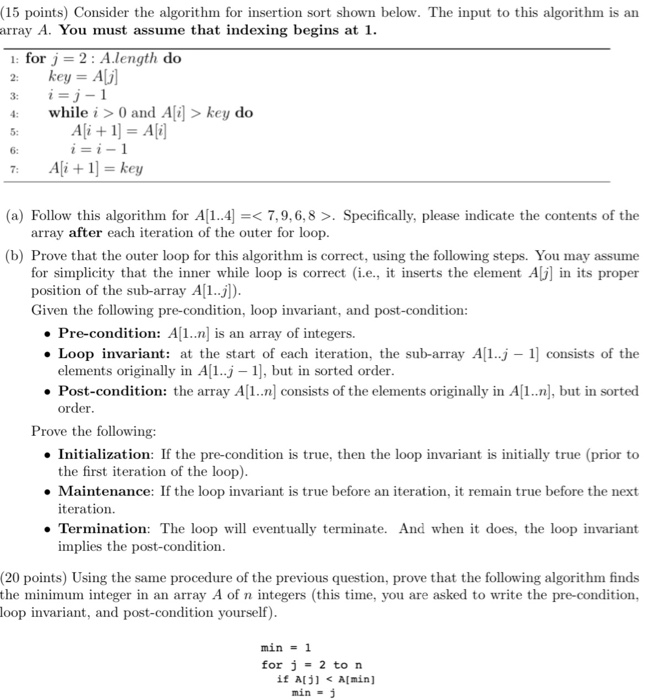(15 points) Consider the algorithm for insertion sort shown below. The input to this algorithm is an earray A. You must assume that indexing begins at 1. 1: for j = 2: A.length do key = A i=j-1 while i > 0 and A[i] > key do Ali + 1] = Ai i=i-1 7: A[i+1] = key (a) Follow this algorithm for A[1..4) =< 7,9,6,8 >. Specifically, please indicate the contents of the array after each iteration of the outer...